# What You Should Know About Differentiation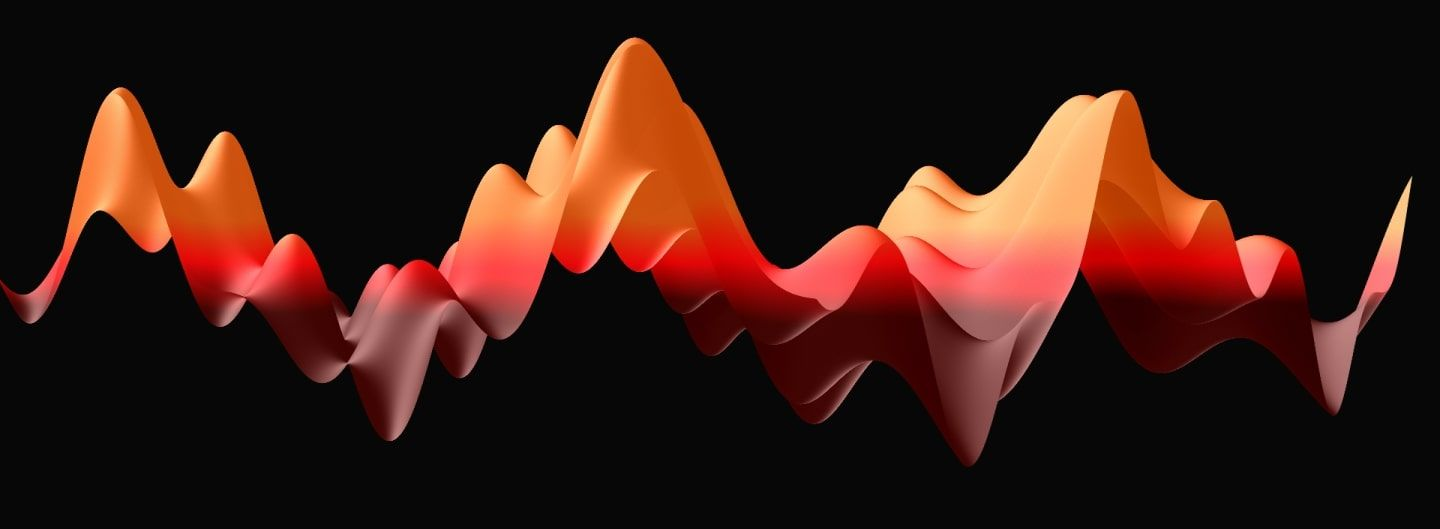In the history of science, few milestones are as significant as inventing the wheel. Even among these, differentiation is a highlight: with calculus, Newton essentially created modern mechanics as we know it. Differentiation enables space travel, function optimization, or even epidemiological models. In machine learning, derivatives are key for training deep neural networks.

However, its importance is not obvious from the mathematical definition: if $f: \mathbb{R} \to \mathbb{R}$ is an arbitrary function, it is said to be differentiable at $x$ if the limit

$f^\prime(x) = \lim_{y \to x} \frac{f(x) - f(y)}{x - y}$

exists, which is called its derivative. This definition is well known but loaded with concepts that are often left unexplained. In this post, our goal is to understand what the derivative really is, how to extend the it to multiple variables, and how it allows us to build models of the world around us. Let's get to it!

## Differentiation as the rate of change

Instead of jumping back straight into the mathematical definition, let's start our discussion with a straightforward example: a point-like object moving along a straight line. The straight line can be modelled with the real numbers $\mathbb{R}$, so it makes sense to describe the motion of our object with the function $f(t): \mathbb{R} \to \mathbb{R}$, mapping a point in time $t$ to a position $f(t)$. Something like this below.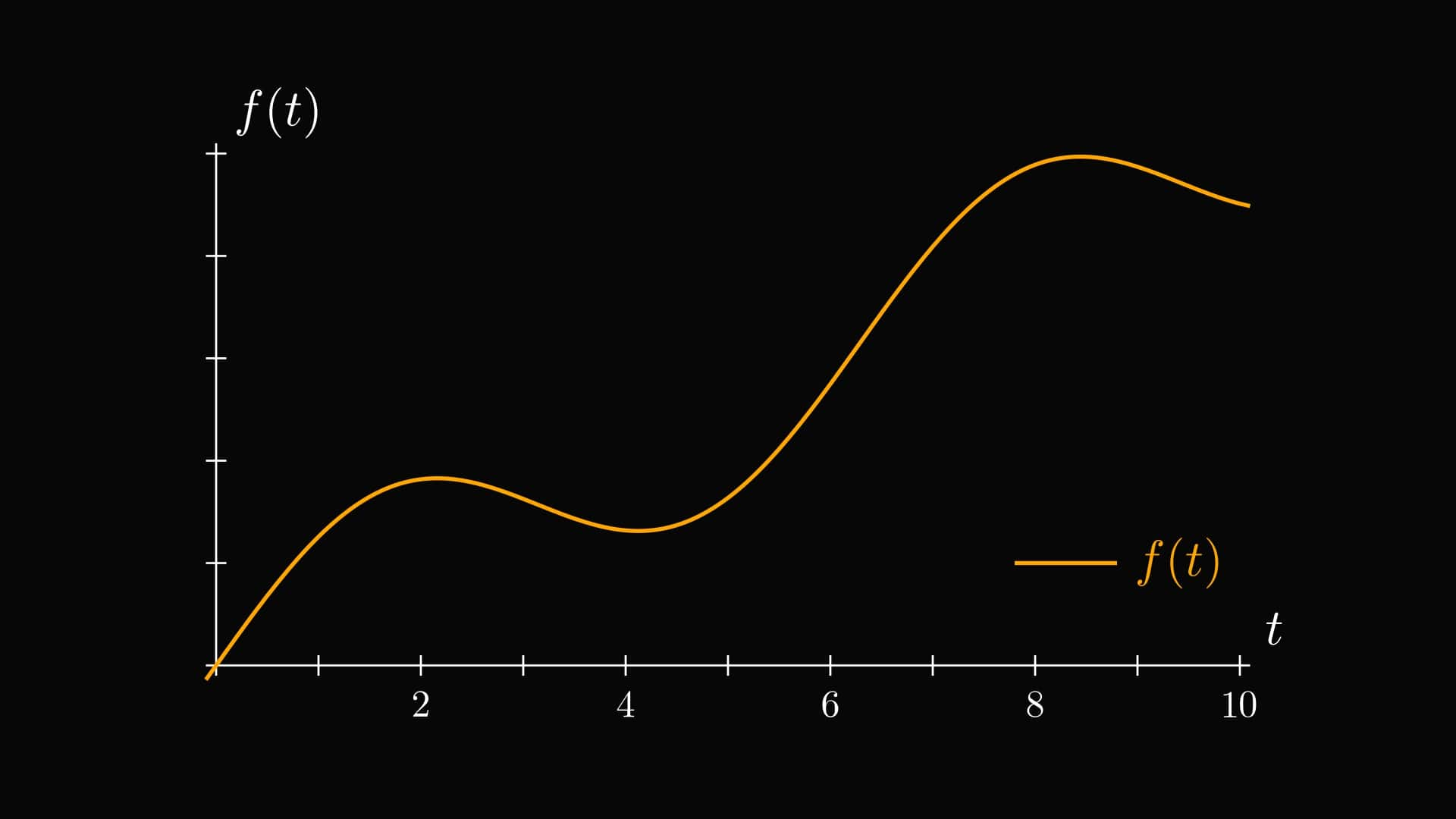Our goal is to calculate the object's speed at a given time. In high school, we learned that

$\text{average speed} = \frac{\text{distance}}{\text{time}}.$

To put this into a quantitative form, if $t_1 < t_2$ are two arbitrary points in time, then

$\text{average speed between } t_1 \text{ and } t_2 = \frac{f(t_2) - f(t_1)}{t_2 - t_1}.$

Expressions like $\frac{f(t_2) - f(t_1)}{t_2 - t_1}$ are called differential quotients. Note that if the object moves backwards, the average speed is negative.

The average speed has a simple geometric interpretation. If you replace the object's motion with a constant velocity motion moving at its average speed, you'll end up at the same place. In graphical terms, this is equivalent of connecting $(t_1, f(t_1))$ and $(t_2, f(t_2))$ with a single line. The average speed is just the slope of this line, as you can see below.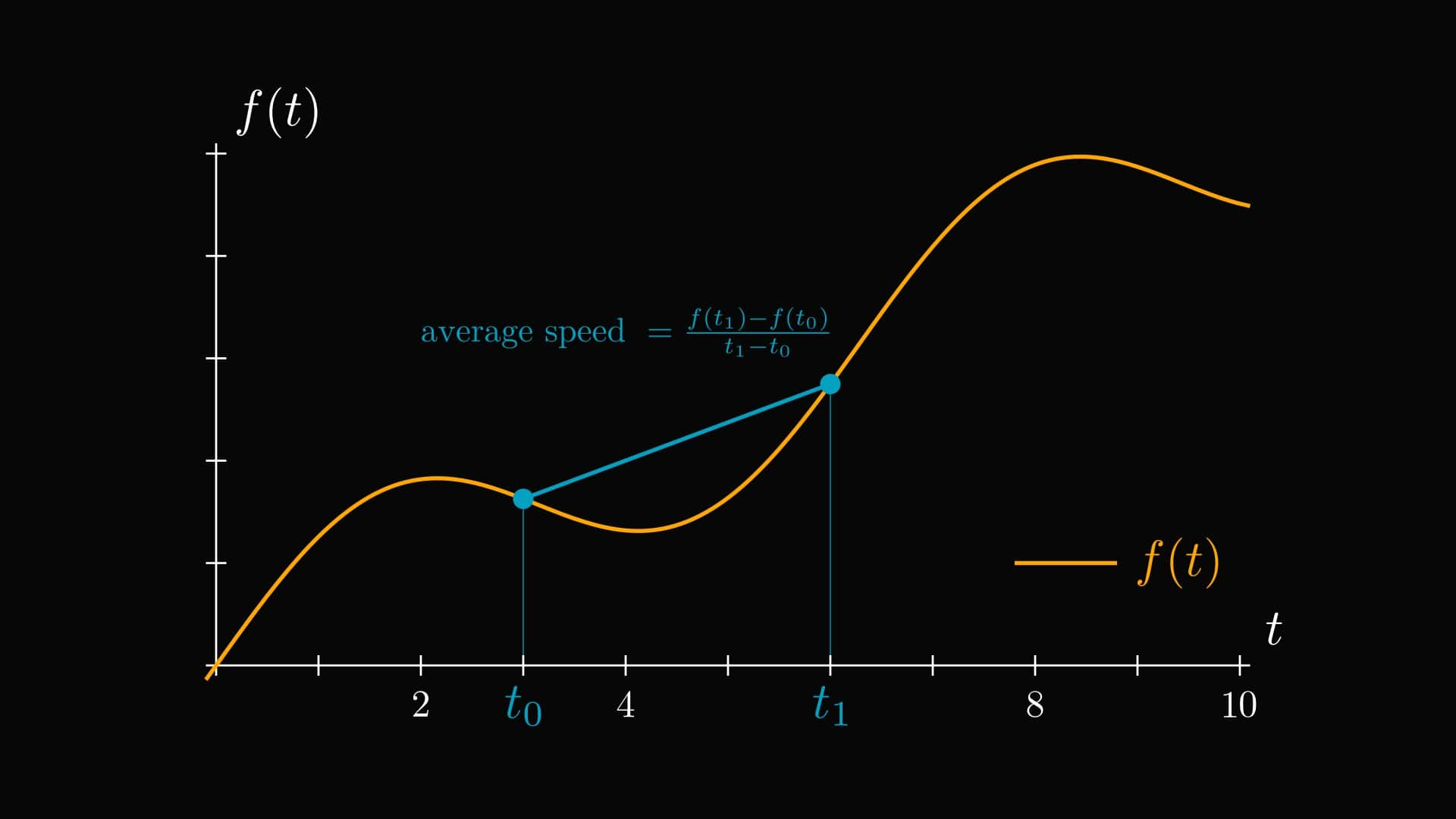Given this, we can calculate the exact speed at a single time point $t$, which we'll denote with $v(t)$. ($v$ is short for velocity.) The idea is simple: the average speed in the small time-interval between $t$ and $t + \Delta t$ should get closer and closer to $v(t)$ if $\Delta t$ is small enough. ($\Delta t$ can be negative as well.)

So,

$v(t) = \lim_{\Delta t \to 0} \frac{f(t + \Delta t) - f(t)}{\Delta t},$

if the above limit exists.

Following our geometric intuition, we can notice that $v(t)$ is simply the slope of the tangent line of $f$ at $t$. This can be beautifully illustrated when we visualize the ratio $\frac{f(t + \Delta t) - f(t)}{\Delta t}$ for a few $\Delta t$-s.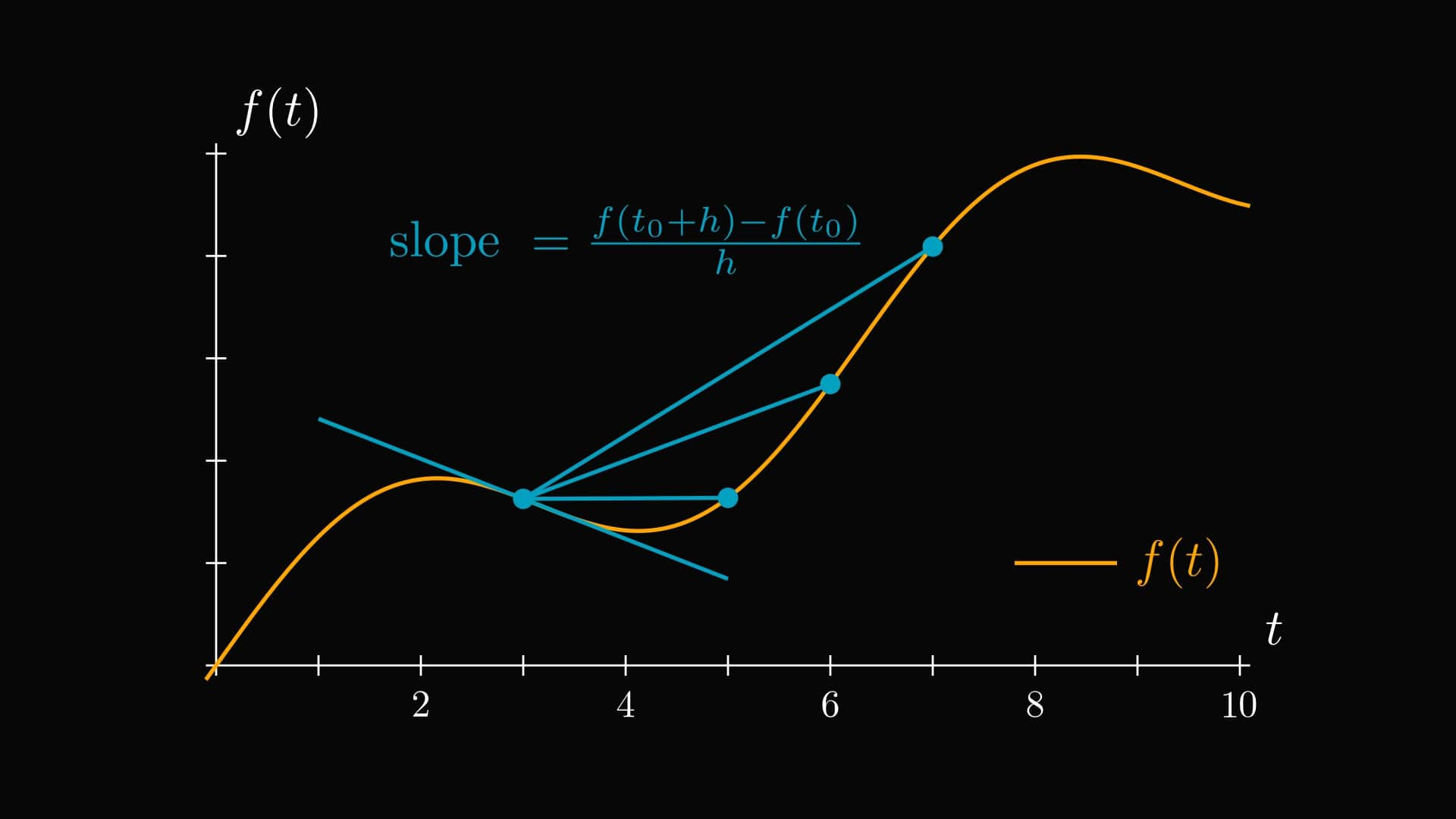Keeping this in mind, we are ready to introduce the formal definition. (The one that we mentioned earlier, but it actually makes sense this time.)

Definition. (Differentiability.) Let $f: \mathbb{R} \to \mathbb{R}$ be an arbitrary function. We say that $f$ is differentiable at $x_0 \in \mathbb{R}$ if the limit

$\frac{df}{dx}(x_0) = \lim_{x \to x_0} \frac{f(x_0) - f(x)}{x_0 - x}$

exists. If so, $\frac{df}{dx}(x_0)$ is called the derivative of $f$ at $x_0$.

In pure English, if $f$ describes a time-distance function of a moving object, then the derivative is simply its speed. In other words, the derivative quantifies the rate of change. Note that differentiability is a property of the function $f$ and the point $x_0$. As we shall see later, some functions are differentiable at some points but not differentiable at others.

Don't let the change in notation from $t$ and $t + \Delta t$ to $x_0$ and $x$ confuse you, this means exactly the same as before. Speaking of confusion, sometimes, the multiple notations for differentiation can be difficult to interpret. For instance, $x$ can denote the variable of $f$ and the exact point where the derivative is taken. To clear this up, here is a quick glossary of terms to clarify the difference between derivative and derivative function.

• $\frac{df}{dx}(x_0)$: derivative of $f$ with respect to the variable $x$ at the point $x_0$. This is a scalar, also denoted with $f^\prime(x_0)$.
• $\frac{df}{dx}$: derivative function of $f$ with respect to the variable $x$. This is a function, also denoted with $f^\prime$.

## Differentiability is smoothness

Now that we understand how derivatives express the rate of change, we'll look at things from a more abstract viewpoint: what does differentiability mean? Mind you, I am not talking about the value of the derivative itself but the fact that it exists. To make my point clear, let's consider two examples.

Example 1. $f(x) = x^2$. Here, we have

\begin{align*} \lim_{y \to x} \frac{f(x) - f(y)}{x - y} &= \lim_{y \to x} \frac{x^2 - y^2}{x - y} \\ &= \lim_{y \to x} \frac{(x - y)(x + y)}{x - y} \\ &= \lim_{y \to x} x + y \\ &= 2x. \end{align*}

So, $f(x) = x^2$ is differentiable everywhere and $f^\prime(x) = 2x$. No surprise here. If you are a visual person, this is how the tangents look.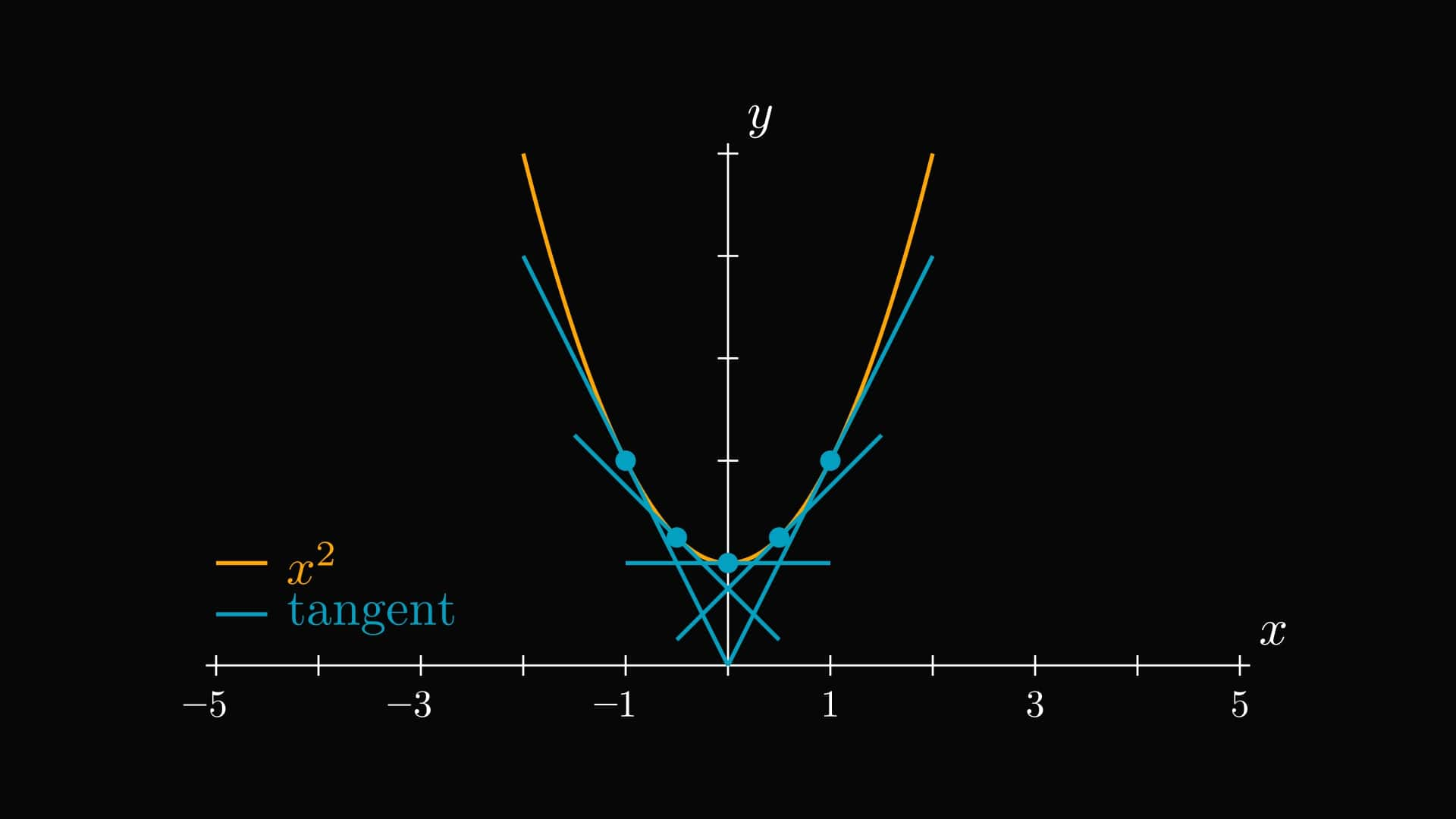The graph of the $x^2$ function is smooth everywhere. However, this is not always the case, leading us to the second example.

Example 2. $f(x) = |x|$ at $x = 0$. For this, we have

\begin{align*} \lim_{y \to 0} \frac{f(0) - f(y)}{0 - y} &= \lim_{y \to 0} \frac{|y|}{y}. \end{align*}

Since

$\frac{|y|}{y} = \begin{cases} 1 & \text{if } y > 0, \\ -1 & \text{if } y < 0, \end{cases}$

this limit does not exist. Thus, $|x|$ is not differentiable at $0$.

It is worth drawing a picture here to enhance our understanding of differentiability. Recall that the value of the derivative at a given point equals the slope of the tangent line to the function's graph. Since $|x|$ has a sharp corner at $0$, the tangent line is not well-defined, as multiple possibilities exist.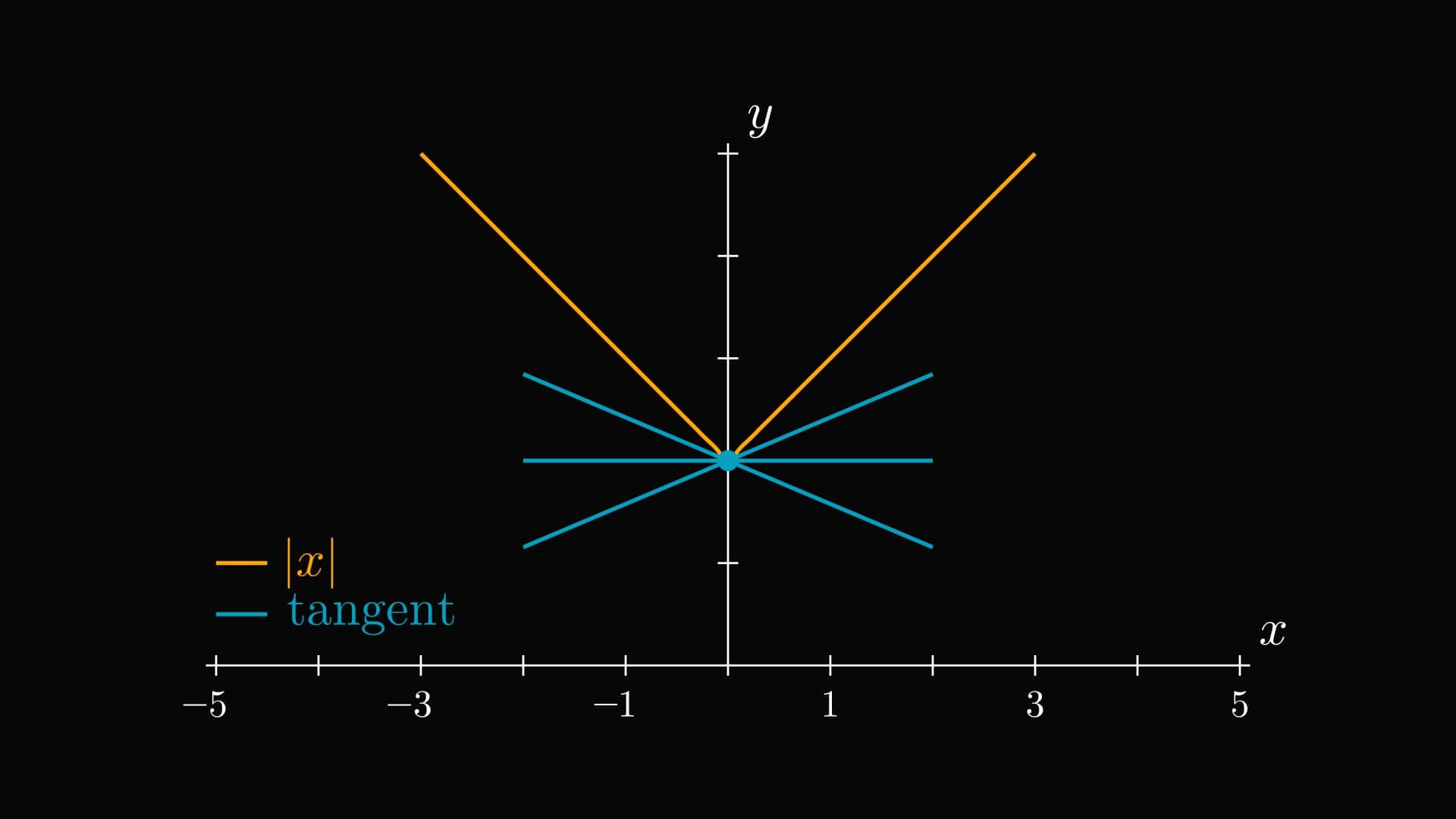In other words, differentiability means no sharp corners in the graph. This is why differentiable functions are often called smooth.

From this perspective, differentiability means manageable behavior: no wrinkles, corners, or sharp changes in value. Next, we'll see an equivalent definition of differentiability involving local approximation with a linear function.

## Differentiation as the best local linear approximation

Do you recall how we introduced the definition of the derivative? Essentially, we approximated the dynamics of a moving point-like object with a constant velocity motion on smaller and smaller time intervals, eventually shrinking down the gap to zero. From the perspective of mechanics, differentiation is the same as swapping the motion with a constant velocity one in a given instant.

We can make this idea mathematically precise with the following theorem. (Yes, a theorem. Don't be scared. Theorems and proofs are just crystallized forms of logically correct statements.)

Theorem. (Differentiation as a local linear approximation.) Let $f: \mathbb{R} \to \mathbb{R}$ be an arbitrary function. The following are equivalent.

(a) $f$ is differentiable at $x_0$.

(b) there is an $\alpha$ such that

$f(x) = f(x_0) + \alpha (x - x_0) + o(|x - x_0|)$

holds as $x \to x_0$.

(Recall that the small O notation means that the function is an order of magnitude smaller around $x_0$ than the function $|x - x_0|$. That is,

$\lim_{x \to x_0} \frac{o(|x - x_0|)}{|x - x_0|} = 0.$

The $\alpha$ in the above theorem is going to be the derivative $f^\prime(x_0)$. In other words, $f(x)$ is locally approximated with the linear function $f(x_0) + f^\prime(x_0) (x - x_0)$.)

Proof. To show the equivalence of two statements, we have to prove that differentiation implies the desired property and vice versa. Although this might seem complicated, it is straightforward and entirely depends on how functions can be written as their limit plus an error term.

(a) $\implies$ (b). The existence of the limit

$\lim_{x \to x_0} \frac{f(x) - f(x_0)}{x - x_0} = f^\prime(x_0)$

implies that we can write the slope of the approximating tangent in the form

$\frac{f(x) - f(x_0)}{x - x_0} = f^\prime(x_0) + \mathrm{error}(x),$

where $\lim_{x \to x_0} \mathrm{error}(x) = 0$. With some simple algebra, we obtain

$f(x) = f(x_0) + f^\prime(x_0)(x - x_0) + \mathrm{error}(x)(x-x_0).$

Since the error term tends to zero as $x$ goes to $x_0$, $\mathrm{error}(x)(x-x_0) = o(|x - x_0|)$, which is what we wanted to show.

(b) $\implies$ (a). Now, repeat what we did in the previous part, just in reverse order. We can rewrite

$f(x) = f(x_0) + \alpha (x - x_0) + o(|x - x_0|)$

in the form

$\frac{f(x) - f(x_0)}{x - x_0} = \alpha + o(1),$

which, according to what we have used before, implies that

$\lim_{x \to x_0} \frac{f(x) - f(x_0)}{x - x_0} = \alpha.$

So, $f$ is differentiable at $x_0$ and its derivative is $f^\prime(x_0) = \alpha$. $\square$

Notice that in the $x$ variable, the expression $f(x_0) + f^\prime(x_0) (x - x_0)$ defines a linear function. In fact, this is the equation of the tangent line! The expression

$f(x) = f(x_0) + \alpha (x - x_0) + o(|x - x_0|)$

tells us that around $x_0$, $f$ equals a linear function plus a small error. You might ask, why is this good for us? For one, this form will work in higher dimensions, as opposed to the limit of differential quotients. Let's take a look!

## Derivatives of multivariable functions

For a single variable function, we defined the derivative as the limit of difference quotients

$\lim_{y \to x} \frac{f(x) - f(y)}{x - y},$

where $x$ and $y$ are real numbers. For a multivariable function $f: \mathbb{R}^n \to \mathbb{R}$, the difference quotients are not defined. Why? Because division with the vector $x - y$ doesn't make sense.

To see what we can do here, let's build our intuition using functions of two variables. (That is, those that are defined on the plane.) In this case, the graph is a surface. For example,

$f(x, y) = \cos(3x + 2y) + \cos(2x + 4y) - 2\sin(x + y)$

looks like this below.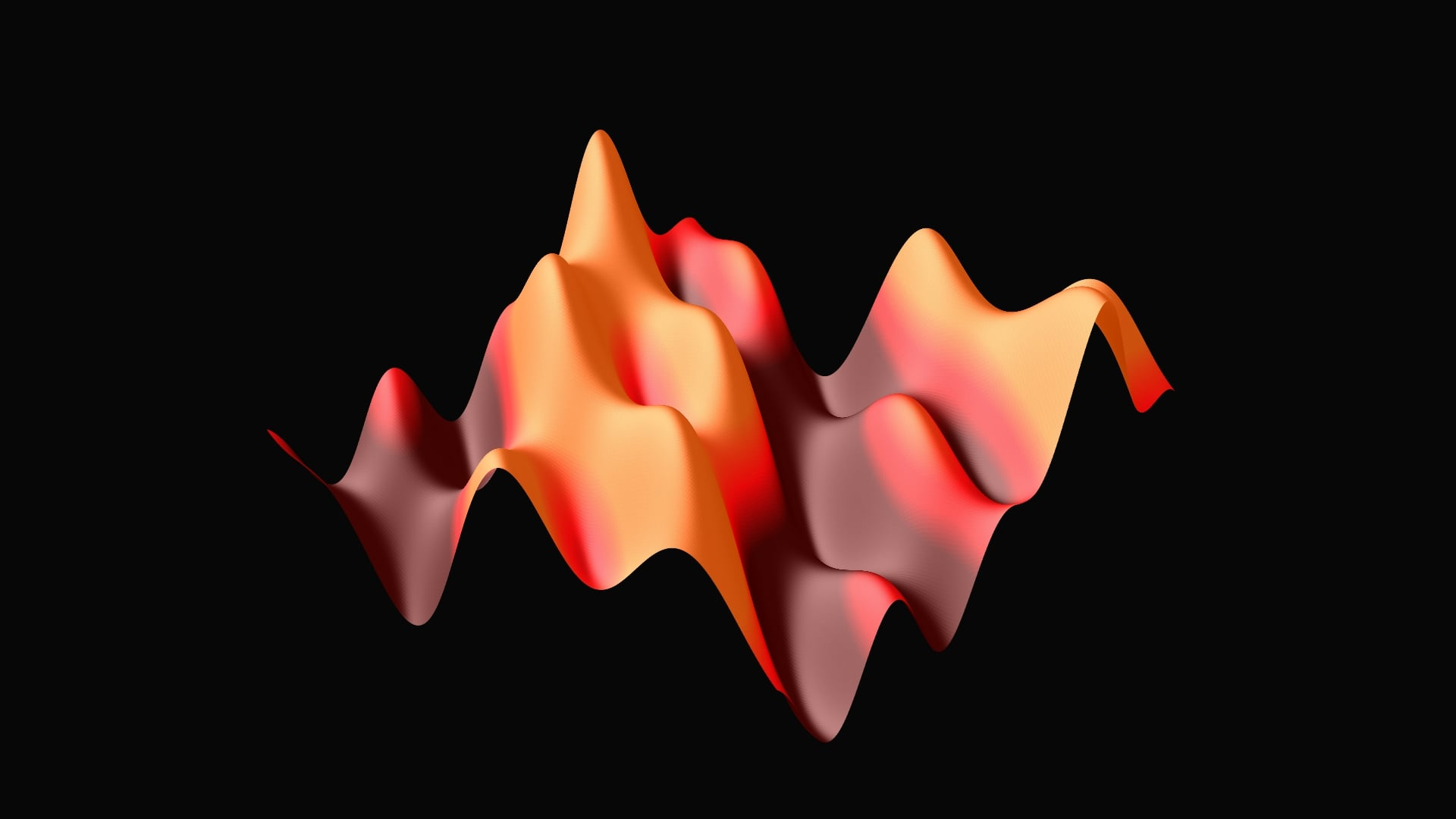We immediately see that the concept of the tangent line is not well defined since we have many tangent lines to a given point on the surface. In fact, we have a whole plane of them, but more on those later. This is called the tangent plane.However, this tangent plane contains two special directions. Suppose we are looking at the tangent plane at $(0, 0)$. For every multivariable function, fixing all but one variable is a function of a single variable. In our case, we would have

$f(x, 0) = \cos(3x) + \cos(2x) - 2\sin(x)$

and

$f(0, y) = \cos(2y) + \cos(4y) - 2\sin(y).$

We can visualize these functions by slicing the surface with a vertical plane perpendicular to one of the axes. Where the plane and the surface meet is the graph of $f(x, 0)$ or $f(0, y)$, depending on which plane you use. This is how it looks.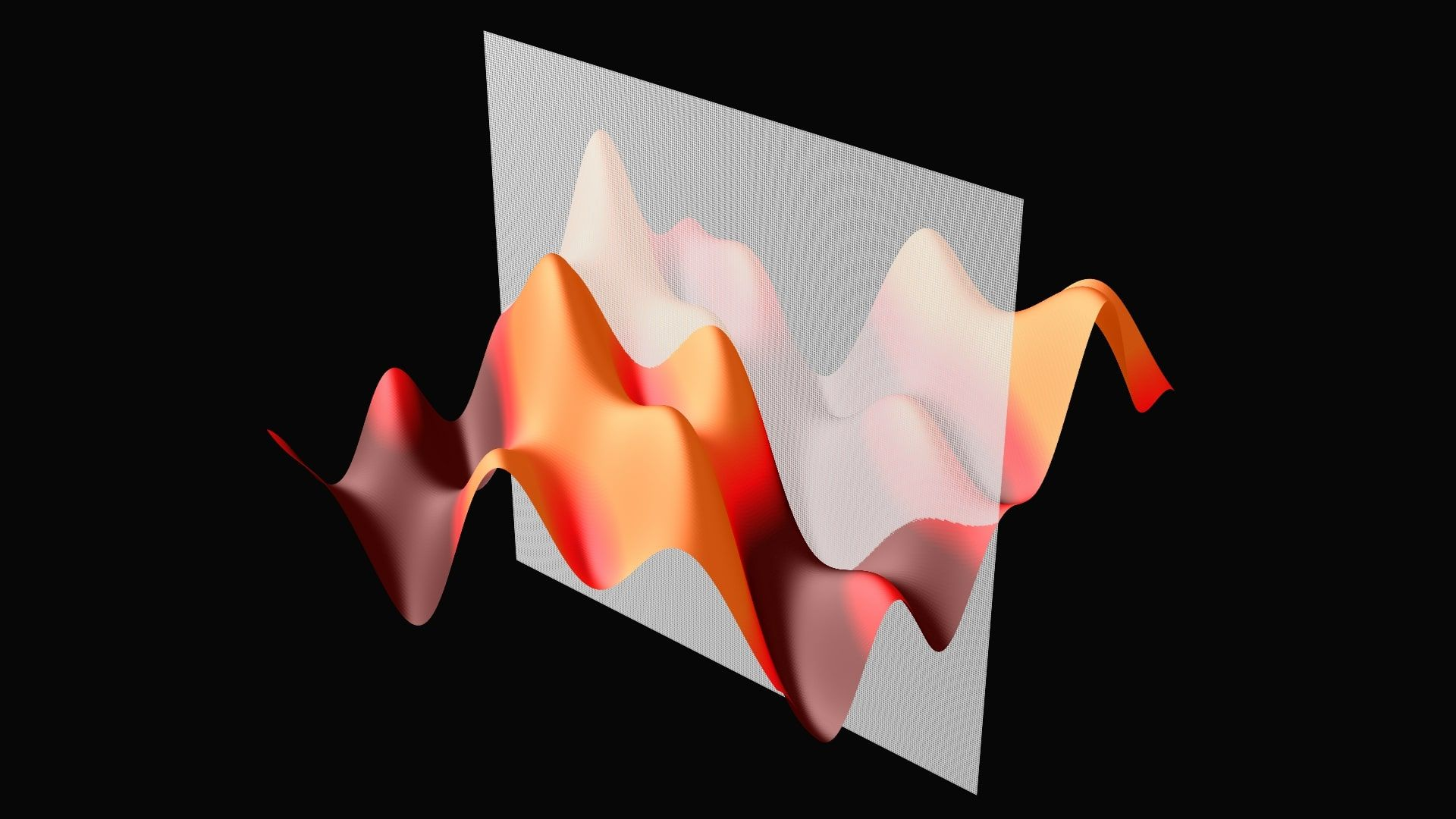We can define the derivatives as we have done in the previous section for these functions. These are called partial derivatives and they play an essential role in generalizing our peak finding algorithm. To formalize it mathematically, they are defined by

\begin{align*} \frac{\partial f(x, y)}{\partial x} &= f_x(x, y) = \lim_{x_0 \to x} \frac{f(x, y) - f(x_0, y)}{x - x_0}, \\ \frac{\partial f(x, y)}{\partial y} &= f_y(x, y) = \lim_{y_0 \to y} \frac{f(x, y) - f(x, y_0)}{y - y_0}. \end{align*}

The values of partial derivatives are the slopes of the tangent plane in the direction parallel with $x$ or the $y$ axis. The direction of the steepest ascent is given by the gradient, defined by

$\nabla f(x, y) = \bigg( \frac{\partial f(x, y)}{\partial x}, \frac{\partial f(x, y)}{\partial y} \bigg).$

(If you are familiar with the famous gradient descent optimization algorithm, this is why the gradient determines the direction of the step.)

## Differentiation as a local linear approximation, revisited

So, instead of having a derivative, we have one for each variable. Can we find a pattern that meaningfully relates all of these partial derivatives to each other? Yes, and this is where the already familiar linear approximations come into the picture. Recall that for a differentiable univariate function $f: \mathbb{R} \to \mathbb{R}$, we have

$f(x) = f(x_0) + \alpha (x - x_0) + o(|x - x_0|),$

and this is going to be the key to defining the analogue of differentiability.

Definition. (Differentiability in multiple variables.) Let $f: \mathbb{R}^n \to \mathbb{R}$ be an arbitrary multivariable function. $f$ is differentiable at $x_0 \in \mathbb{R}^n$ if there exists a $\nabla f(x_0) \in \mathbb{R}^n$ such that

$f(x) = f(x_0) + \nabla f(x_0) \cdot (x - x_0) + o(| x - x_0 |)$

holds, where $x \cdot y$ denotes the dot product of the vectors $x, y \in \mathbb{R}^n$. $\nabla f(x_0)$ is called the gradient of $f$ at $x_0$.

This example shows the importance of looking at mathematical objects from several different directions. Sometimes, an alternate viewpoint can help to extend the scope of definitions significantly. Just like differentiation and the best linear approximation.